Next: Building additional iterators Up: Partial inverse heuristic for Previous: Evaluation by simulation.

# Convergence of partial inverses

The question of convergence of the different iterators can be partially answered. For functions with two occurrences of the unknown,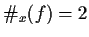, in general either one or the other derived iterator converges. This is proven as follows.

Let f(x1,x2)=0 be the equation to be solved, identifying each occurrence of x by x1 and x2. Let Fi(x) be the iterator derived from inverting f on xi. Formally this means that

f(F1(x),x) = 0

and

f( x, F2(x) ) = 0

Let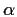be a root of f,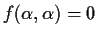. The convergence of the iterators depends on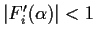. By computing derivatives with respect to x of the above identities, we find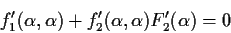For this system of equations to have a non-null solution in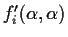, its determinant must be zero, or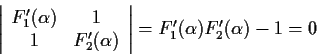and from this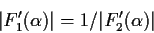So unless both absolute values are equal to one, then one iterator converges while the other diverges. This is a very encouraging result, it guarantees that one of the iterators will succeed for each of the roots.

For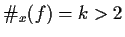the results are much weaker. For general x, the condition becomes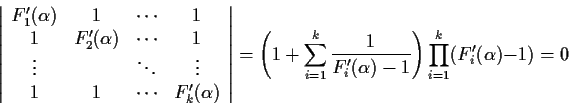Then, if k-1 of the values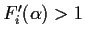, the remaining one must be between 0 and 1 and hence the corresponding iterator converges. This does not guarantee convergence for every case, as all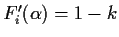, i.e.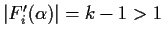is also a possible solution and in this case all iterators will diverge.

The above theorem for two occurrences, strongly suggests that grouping variables (if possible) while building the iterators, is a good strategy.Next: Building additional iterators Up: Partial inverse heuristic for Previous: Evaluation by simulation.
Gaston Gonnet
1998-07-08